Python  - Panda - Statistics                               Home : www.sharetechnote.com

Panda - Statistics

In this page, I will give you some of the basic operations for Panda. I will not go very deep into Panda functions in this page. This page is just for you to try some basic things without getting scared of too complicated operation right after you installed panda package.

The data for this tutorial is from https://support.spatialkey.com/spatialkey-sample-csv-data/ (If this link does not work, try this).

Download the file, unzip it and put it into the folder where Python is installed. Of course, you can put the file into other places. However, if you want to run the command in this tutorial as it is without modifying the data path, put the csv file in your Python installation folder.

The data structure of the csv file is as shown below.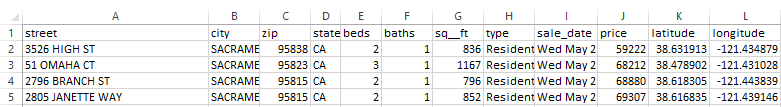Following is the list of all the commands used in this page. These procedure is based on the tutorial (very useful) A Complete Tutorial to Learn Data Science with Python from Scratch (I would recommend you to read this document. He posted many other useful tutorials as well). I just used different data set and with some modification for my own experiment.

 panda_stat01.py import sys import pandas as pd import numpy as np import matplotlib import matplotlib.pyplot as plt import random as rd   print('Python: {}'.format(sys.version)) print('pandas: {}'.format(pd.__version__)) print('numpy: {}'.format(np.__version__)) print('matplotlib {}'.format(matplotlib.__version__))   df = pd.read_csv('Sacramentorealestatetransactions.csv')   print(df.head(10))   print(df.describe())   df_NumData = df[['beds','baths','sq__ft','price']] print(df_NumData.describe())   df['price'].hist(bins=100) plt.show()   df.boxplot(column='price') plt.show()   df.boxplot(column='price', by = 'beds') plt.show()   df[['price','beds']].groupby('beds').boxplot() plt.show()   bedcount = df['beds'].value_counts() print(bedcount)   bedcount = df['beds'].value_counts(ascending=True) print(bedcount)   print(bedcount.sort_index(axis=0,ascending=True))   print(bedcount.sort_index(axis=0,ascending=False))   print(bedcount.sort_values(axis=0))   avgPricePivot = df.pivot_table(values='price',index=['beds'],aggfunc=lambda x: x.mean()) print(avgPricePivot)   avgPricePivot = df.pivot_table(values='price',index=['beds'],aggfunc=lambda x: [x.mean(),x.min(), x.max()]) print(avgPricePivot)   pd.plotting.scatter_matrix(df_NumData) plt.show()

I will break down the file shown above into each step as listed below and put descriptions on it.

import libraries

Followings are all the packages (libaries) that I need in this tutorial.

import sys

import pandas as pd

import numpy as np

import matplotlib

import matplotlib.pyplot as plt

import random as rd

Checking the version of Packages

This is not a mandatory step, but just to show you the version of each packages that I am using I put these lines. Some of the command (function) in this tutorial may not work properly or work but with some warning depending on the version that you are using.

print('Python: {}'.format(sys.version))

print('pandas: {}'.format(pd.__version__))

print('numpy: {}'.format(np.__version__))

print('matplotlib {}'.format(matplotlib.__version__))

Following is the result of the execution on my system at the time of writing this tutorial.

Python: 3.6.2 (v3.6.2:5fd33b5, Jul  8 2017, 04:14:34) [MSC v.1900 32 bit (Intel)]

pandas: 0.20.3

numpy: 1.13.3

matplotlib 2.1.0

Reading data set from a csv file

I read a data set from a csv file and store it to the variable df. In this example, I put the csv file in the same foder as the python script file that I wrote. That's why I don't put the file path and put the filename only.

Preview of Data Structure

Don't do print(df) unless you really need to do it. It will print out too much data. Instead, you can print out just a few lines from the beginning of the file and see how the overall data structure is as shown below.

Following is the result of this command.

street        city    zip state  beds  baths  sq__ft  \

0      3526 HIGH ST  SACRAMENTO  95838    CA     2      1     836

1       51 OMAHA CT  SACRAMENTO  95823    CA     3      1    1167

2    2796 BRANCH ST  SACRAMENTO  95815    CA     2      1     796

3  2805 JANETTE WAY  SACRAMENTO  95815    CA     2      1     852

4   6001 MCMAHON DR  SACRAMENTO  95824    CA     2      1     797

type                     sale_date  price   latitude   longitude

0  Residential  Wed May 21 00:00:00 EDT 2008  59222  38.631913 -121.434879

1  Residential  Wed May 21 00:00:00 EDT 2008  68212  38.478902 -121.431028

2  Residential  Wed May 21 00:00:00 EDT 2008  68880  38.618305 -121.443839

3  Residential  Wed May 21 00:00:00 EDT 2008  69307  38.616835 -121.439146

4  Residential  Wed May 21 00:00:00 EDT 2008  81900  38.519470 -121.435768

With applying the single function as follows, you can get the basic statistics on every numerical data.

print(df.describe())

Following is the result of the command. This commands automatically check each column on whether it is numerical data or not and calculate the statistics.

zip        beds       baths       sq__ft          price  \

count    985.000000  985.000000  985.000000   985.000000     985.000000

mean   95750.697462    2.911675    1.776650  1314.916751  234144.263959

std       85.176072    1.307932    0.895371   853.048243  138365.839085

min    95603.000000    0.000000    0.000000     0.000000    1551.000000

25%    95660.000000    2.000000    1.000000   952.000000  145000.000000

50%    95762.000000    3.000000    2.000000  1304.000000  213750.000000

75%    95828.000000    4.000000    2.000000  1718.000000  300000.000000

max    95864.000000    8.000000    5.000000  5822.000000  884790.000000

latitude   longitude

count  985.000000  985.000000

mean    38.607732 -121.355982

std      0.145433    0.138278

min     38.241514 -121.551704

25%     38.482717 -121.446127

50%     38.626582 -121.376220

75%     38.695589 -121.295778

max     39.020808 -120.597599

As you see above, this function extract the statistical data from every numerical data regardless of whether it is really meaningful or not. For example, you know that the mean, std, max of the zip code is meaningless but descrbe() function calculate those statistics since the zip code is typed in as a numeric form. If you want to get more meaningful information, extract the numerical data that are meaningful to you and then apply describe() function as below.

df_NumData = df[['beds','baths','sq__ft','price']]

print(df_NumData.describe())

Following is the result of this command.

beds       baths       sq__ft          price

count  985.000000  985.000000   985.000000     985.000000

mean     2.911675    1.776650  1314.916751  234144.263959

std      1.307932    0.895371   853.048243  138365.839085

min      0.000000    0.000000     0.000000    1551.000000

25%      2.000000    1.000000   952.000000  145000.000000

50%      3.000000    2.000000  1304.000000  213750.000000

75%      4.000000    2.000000  1718.000000  300000.000000

max      8.000000    5.000000  5822.000000  884790.000000

Plotting a Histogram

You can plot a Histogram for a data series as shown below.

df['price'].hist(bins=100)

plt.show()

Following is the result of this command.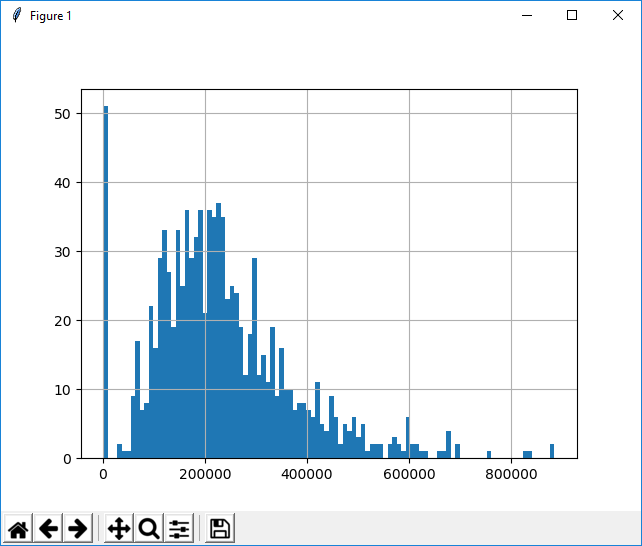Graphing a Boxplot

You can easily draw a box plot for a specific column or a dataset using boxplot() function as shown below. (Regarding the interpretation of the box plot, refer to the tutorial RANGE, INTERQUARTILE RANGE AND BOX PLOT . This is not my note, but much better written than I could).

df.boxplot(column='price')

plt.show()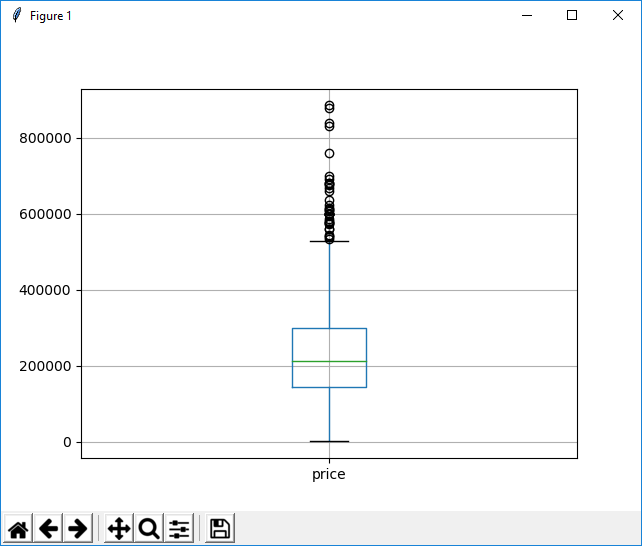df.boxplot(column='price', by = 'beds')

plt.show()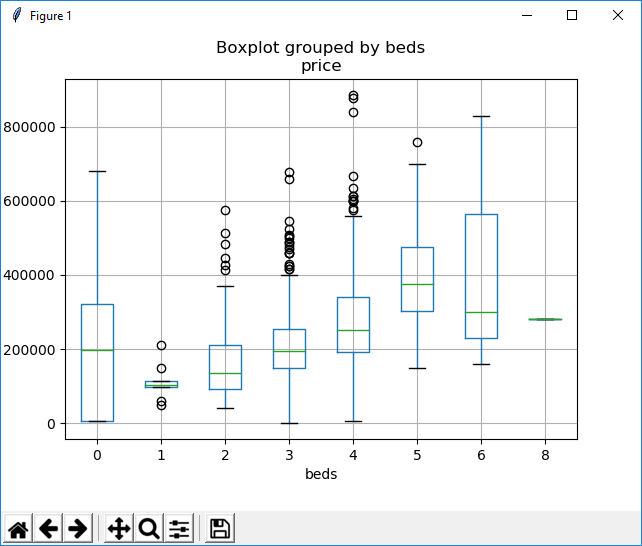NOTE : I got following warning on my system (Python 3.62 on Windows 10). So this command may not work any more if you are using later than Python 3.62.

Warning (from warnings module):

File "C:\Users\ca090406\AppData\Local\Programs\Python\Python36-32\lib\site-packages\numpy\core\fromnumeric.py", line 57

return getattr(obj, method)(*args, **kwds)

FutureWarning: reshape is deprecated and will raise in a subsequent release. Please use .values.reshape(...) instead

Getting the Data Count

You can extract a certain colum and count the number of data for each different items in the columns as follows.

bedcount = df['beds'].value_counts()

print(bedcount)

Following is the result of this commands (The number on the left represents the number of beds and the number on the right represents the total number of occurrence of each number of beds in the data).

3    413

4    258

2    133

0    108

5     59

1     10

6      3

8      1

Name: beds, dtype: int64

You can change the order of the data by specifying 'ascending' parameter as shown below.

bedcount = df['beds'].value_counts(ascending=True)

print(bedcount)

Following is the result of this commands

8      1

6      3

1     10

5     59

0    108

2    133

4    258

3    413

Name: beds, dtype: int64

Sorting the data

You can sort a data set using the function sort_index() or sort_value() as shown below.

bedcount = df['beds'].value_counts()

print(bedcount.sort_index(axis=0,ascending=True))

0    108

1     10

2    133

3    413

4    258

5     59

6      3

8      1

Name: beds, dtype: int64

print(bedcount.sort_index(axis=0,ascending=False))

8      1

6      3

5     59

4    258

3    413

2    133

1     10

0    108

Name: beds, dtype: int64

print(bedcount.sort_values(axis=0))

8      1

6      3

1     10

5     59

0    108

2    133

4    258

3    413

Name: beds, dtype: int64

Generating a Pivot Table

Probably one of the most powerful function in pandas would be pivot_table() function. If you have no experience of generating Pivot table or don't know what is a Pivot Table, I would suggest you try to generate some of pivot tables using Spreadsheet program (like Microsoft Excel). I would explain the usage of pivot_table() function in comparison to Microsoft Excel Pivot Table as shown below.

Example 1 >  Number of bed vs average price

avgPricePivot = df.pivot_table(values='price',index=['beds'],aggfunc=lambda x: x.mean())

print(avgPricePivot)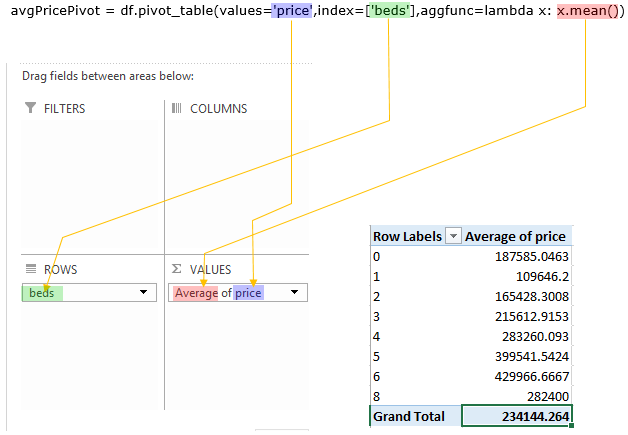Following is the result of the execution shown above.

price

beds

0     187585.046296

1     109646.200000

2     165428.300752

3     215612.915254

4     283260.093023

5     399541.542373

6     429966.666667

8     282400.000000

Example 2 > Number of Bed vs [mean, min, max price]

You can specify the multiple columns in the pivot table by modifying the lamda function as shown below.

avgPricePivot = df.pivot_table(values='price',index=['beds'],

aggfunc=lambda x: [x.mean(),x.min(), x.max()])

print(avgPricePivot)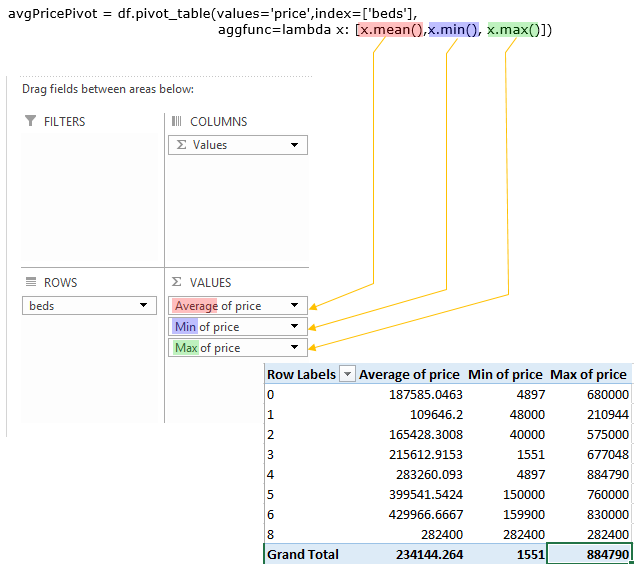Following is the result of the execution shown above.

price

beds

0       [187585.046296, 4897, 680000]

1           [109646.2, 48000, 210944]

2      [165428.300752, 40000, 575000]

3       [215612.915254, 1551, 677048]

4       [283260.093023, 4897, 884790]

5     [399541.542373, 150000, 760000]

6     [429966.666667, 159900, 830000]

8          [282400.0, 282400, 282400]

Example 3 > City vs Available Bed Count

This is not directly related to pivot table function, but I ran this first so that the every column gets printed out for display.

>>> pd.set_option('display.max_columns', None)

Now create the pivot table showing City Name in rows, the number of beds in column and the number of available units in each cell.

>>> BedCountInCity = df.pivot_table(values='price',index=['city'],columns=['beds'],aggfunc=lambda x: x.count())

Just in canse the result values has NaN, replace the NaN with 0 and convert the values in integer.

>>> BedCountInCity = BedCountInCity.fillna(0).astype(int)

Print the first 5 rows of the data.

beds            0  1  2   3   4  5  6  8

city

ANTELOPE        0  0  3  18   9  3  0  0

AUBURN          0  0  2   1   2  0  0  0

CAMERON PARK    1  0  3   4   1  0  0  0

CARMICHAEL      0  0  4  11   5  0  0  0

CITRUS HEIGHTS  0  0  4  20  10  1  0  0

Example 4 > City vs Average Prices for different number of beds (Unit Size)

This is not directly related to pivot table function, but I ran this first so that the every column gets printed out for display.

>>> pd.set_option('display.max_columns', None)

Now create the pivot table showing City Name in rows, the number of beds in column and the number of available units in each cell.

>>> AveragePricePerUnit = df.pivot_table(values='price',index=['city'],columns=['beds'],aggfunc=lambda x: x.mean())

Just in canse the result values has NaN, replace the NaN with 0 and convert the values in integer.

>>> AveragePricePerUnit = AveragePricePerUnit.fillna(0).astype(int)

Print the first 5 rows of the data.

beds                 0  1       2       3       4       5  6  8

city

ANTELOPE             0  0  152083  207754  279617  320000  0  0

AUBURN               0  0  272500  504000  490227       0  0  0

CAMERON PARK    119000  0  206666  312375  423000       0  0  0

CARMICHAEL           0  0  284209  257499  388873       0  0  0

CITRUS HEIGHTS       0  0  112562  192702  198867  256054  0  0

Graphing the ScatterMatrix

When you have a dataset with multiple numerical columns, you can visualize the correlations among those columns by drawing scatterplots between each of the items. you can do this very easily as shown below.

df_NumData = df[['beds','baths','sq__ft','price']]

pd.plotting.scatter_matrix(df_NumData)

plt.show()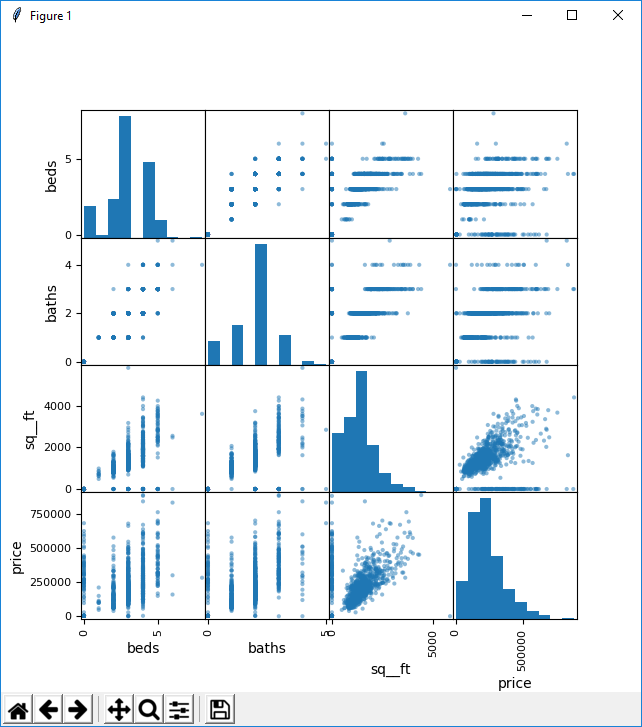NOTE : If the command shown above does not work on your system because you are using older version of the package, you may try as follows.

df_NumData = df[['beds','baths','sq__ft','price']]

pd.tools.plotting.scatter_matrix(df_NumData)

plt.show()

Regarding the interpretation of the scatter plot, I would suggest you to go through CONTINGENCY TABLE, SCATTERPLOT & PEARSON’S R

Reference :